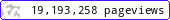•••••MadAsMaths.com :: I.Y.G.B. Practice Papers :: Core Practice Papers 2005 Spec :: C1 Practice Papers

I.Y.G.B. C1 PRACTICE PAPERS

These papers have been written recently and follow the Pearson/Edexcel Syllabus introduced in 2005. The students that have used these papers feel that they are slightly harder than the actual examination papers.

• Papers A to R have standard difficulty with later papers usually more difficult.

• Papers S and T are extremely hard.

• Papers U to Z are hard.

All papers have model solutions and most have guide marking schemes.

The difficulty rating (the second number indicated at the front of each paper) is a very rough UMS converter, so long as the total marks in the paper are 45 or more.

UMS = TOTAL MARKS multiplied by the DIFFICULTY RATING
e.g. total marks = 55
difficulty rating = 1.4033
U.M.S = 77 (approx).

 c1_a.pdfc1_a_marks.pdfc1_a_solutions.pdfc1_b.pdfc1_b_marks.pdfc1_b_solutions.pdfc1_c.pdfc1_c_marks.pdfc1_c_solutions.pdfc1_d.pdfc1_d_marks.pdfc1_d_solutions.pdfc1_e.pdfc1_e_marks.pdfc1_e_solutions.pdfc1_f.pdfc1_f_marks.pdfc1_f_solutions.pdfc1_g.pdfc1_g_marks.pdfc1_g_solutions.pdfc1_h.pdfc1_h_marks.pdfc1_h_solutions.pdfc1_i.pdfc1_i_marks.pdfc1_i_solutions.pdfc1_j.pdfc1_j_marks.pdfc1_j_solutions.pdfc1_k.pdfc1_k_marks.pdfc1_k_solutions.pdfc1_l.pdfc1_l_marks.pdfc1_l_solutions.pdfc1_m.pdfc1_m_marks.pdfc1_m_solutions.pdfc1_n.pdfc1_n_marks.pdfc1_n_solutions.pdfc1_o.pdfc1_o_marks.pdfc1_o_solutions.pdfc1_p.pdfc1_p_marks.pdfc1_p_solutions.pdfc1_q.pdfc1_q_marks.pdfc1_q_solutions.pdfc1_r.pdfc1_r_marks.pdfc1_r_solutions.pdfc1_s.pdfc1_s_marks.pdfc1_s_solutions.pdfc1_t.pdfc1_t_marks.pdfc1_t_solutions.pdfc1_u.pdfc1_u_marks.pdfc1_u_solutions.pdfc1_v.pdfc1_v_marks.pdfc1_v_solutions.pdfc1_w.pdfc1_w_marks.pdfc1_w_solutions.pdfc1_x.pdfc1_x_marks.pdfc1_x_solutions.pdfc1_y.pdfc1_y_marks.pdfc1_y_solutions.pdfc1_z.pdfc1_z_marks.pdfc1_z_solutions.pdf